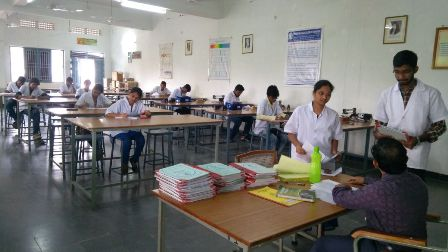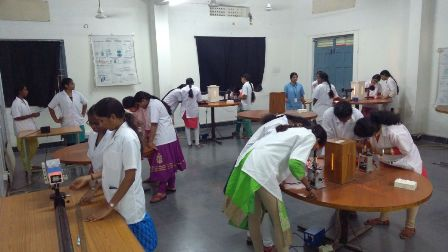### Department Laboratories

#### PHYSICS LAB

Details of Physics Laboratory

The department of Physics has highly dedicated and well qualified, research oriented faculty members. The Physics laboratory caters to the need of scientific pursuits of B. Tech students and is well equipped with modern infrastructure. The laboratory is spacious with experiments like Light Emitting diode, Solar cell, He – Ne Laser source, spectrometers and Micro scopes etc. The department is constantly endeavors to provide a clear understanding of the technical curriculum of engineering students. The faculty aims to train every student for proper exposure in the laboratory experiments.

VISION:

The Freshman Engineering Department is committed to provide quality education to the students through conceptual training in physical sciences empowering them with the analytical and communication skills which enable them to attain their professional and Academic Goals.

MISSION:

• To develop a strong conceptual knowledge with an emphasis on problem solving.

• To produce globally competent graduates having communication skills and behaviour skills.

• Enhance the student’s fundamental knowledge in basics of the physical sciences preparing them for engineering.

• To provide a vibrant academic environment that imparts value addition to faculty and students to achieve personal and professional growth.

List of Major Experiments in Physics lab (R14)

1. Determine the frequency of a electrical tuning fork using Meldy’s apparatus.
2. Determine the frequency of AC supply by using Sonometer.
3. Study the characteristics of L.C.R. Circuit.
4. Study the magnetic field along the axis of a current carrying coil and to verify Stewart’s & Gee’s law.
5. Determine the Refractive index of a given prism.
6. Determine the wavelengths of various spectral lines using grating.
7. Determination of wavelength of Laser radiation.
8. Determine the width of single slit by forming diffraction pattern.
9. Determine the specific rotation of sugar solution by using a Polarimeter.
10. Determine the Radius of Curvature of Plano- Convex lens by forming Newton’s Rings.

ENGINEERING PHYSICS LAB (R17)

List of Experiments

SEM: I

GENERAL EXPERIMENTS:

1. Determine the frequency of AC supply by using Sonometer

2. Determine the frequency of a tuning fork by using Melde's arrangement.

3. Study the characteristics of L.C.R Circuit.

4. Study the magnetic field along the axis of a current carrying circular coil using Stewart’s & Gee’s apparatus and to verify Biot - Savart’s law.

5. Determine the rigidity modulus of a given material using Torsional pendulum.

6. Study the characteristics of Thermister.

7. Determination of time constant of a RC Circuit.

OPTICS LAB EXPERIMENTS:

8. Determine the wavelength and divergence of a laser radiation.

9. Determine the width of a single slit by forming diffraction pattern.

10. Determine the Radius of Curvature of a Plano - Convex lens by forming Newton's Rings.

11. Find the specific rotation of sugar solution by using a polarimeter.

12. Determine the Refractive index of a material of the given prism.

13. Determine the Wavelengths of various spectral lines by using diffraction grating.

14. Determination of a thickness of thin wire by using wedge shaped film.

APPLIED PHYSICS LAB (R17)

List of Experiments

SEM: I & II

GENERAL EXPERIMENTS:

1. Study the characteristics of LED.

2. Determine the energy band gap of a semi conductor Diode.

3. Determine the frequency of AC supply by using Sonometer.

4. Study the characteristics of Zener Diode.

5. Study the magnetic field along the axis of a current carrying circular coil using Stewart’s & Gee’s apparatus and to verify Biot - Savart’s law.

6. Study the characteristics of Solar cell

7. Determine the dielectric constant of a dielectric material.

8. Study the characteristics of Photo diode

OPTICS LAB EXPERIMENTS:

9. Determine the wavelength and divergence of a laser radiation.

10. Determine the width of a single slit by forming diffraction pattern.

11. Determine the Radius of Curvature of a Plano - Convex lens by forming Newton's Rings.

12. Find the specific rotation of sugar solution by using a polarimeter.

13. Determine the Refractive index of a material of the given prism.

14. Determine the Wavelengths of various spectral lines by using diffraction grating.

15. Determination of a thickness of thin wire by using wedge shaped film.students Performing the experiments in Physics laboratoryStudents conducting The Newton's Ring experiment in Optics lab

List of Major Equipment:

 Sl. Name of the laboratory Name of the Equipment Quantity Total Equipment Cost 1 ENGINEERING PHYSICS LAB / APPLIED PHYSICS LAB Single & double slit set with He- Ne Laser Source 02 Rs. 1,13,000/- Spectro Meter 05 Rs. 85,769/- Travelling Microscope 02 Rs.18,579/- C R O 20 MHz 01 Rs. 21,000/- Micro scope Compact form 04 Rs.39,401/- Polarimeter 04 Rs. 32,420/- Diode Laser Source 03 Rs. 15,120/- Stewart and Gee’s  Apparatus 09 Rs. 23,800/- Optical Fiber 02 Rs.8,000/- Digital Function Generator 02 Rs.13,000/- Melde’s Apparatus 05 Rs. 16,486/- Transformers 06 Rs.20,090/- Four Dial resistance box 02 Rs. 6000/- Four Dial Inductance Box 02 Rs. 5,000/- Power supply 20 Rs. 80,961/- Thermistor 03 Rs. 7,090/- Solar Panel 07 Rs. 6,000/- TOTAL EQUIPMENT COST Rs.5,11,716-

#### English lAB

English Laboratory aims at training the students of both UG and PG during odd and even semesters with communication and presentation skills which are considered to be inevitable for seeking employment. It has two parts,

• CALL LAB
• ICS LAB